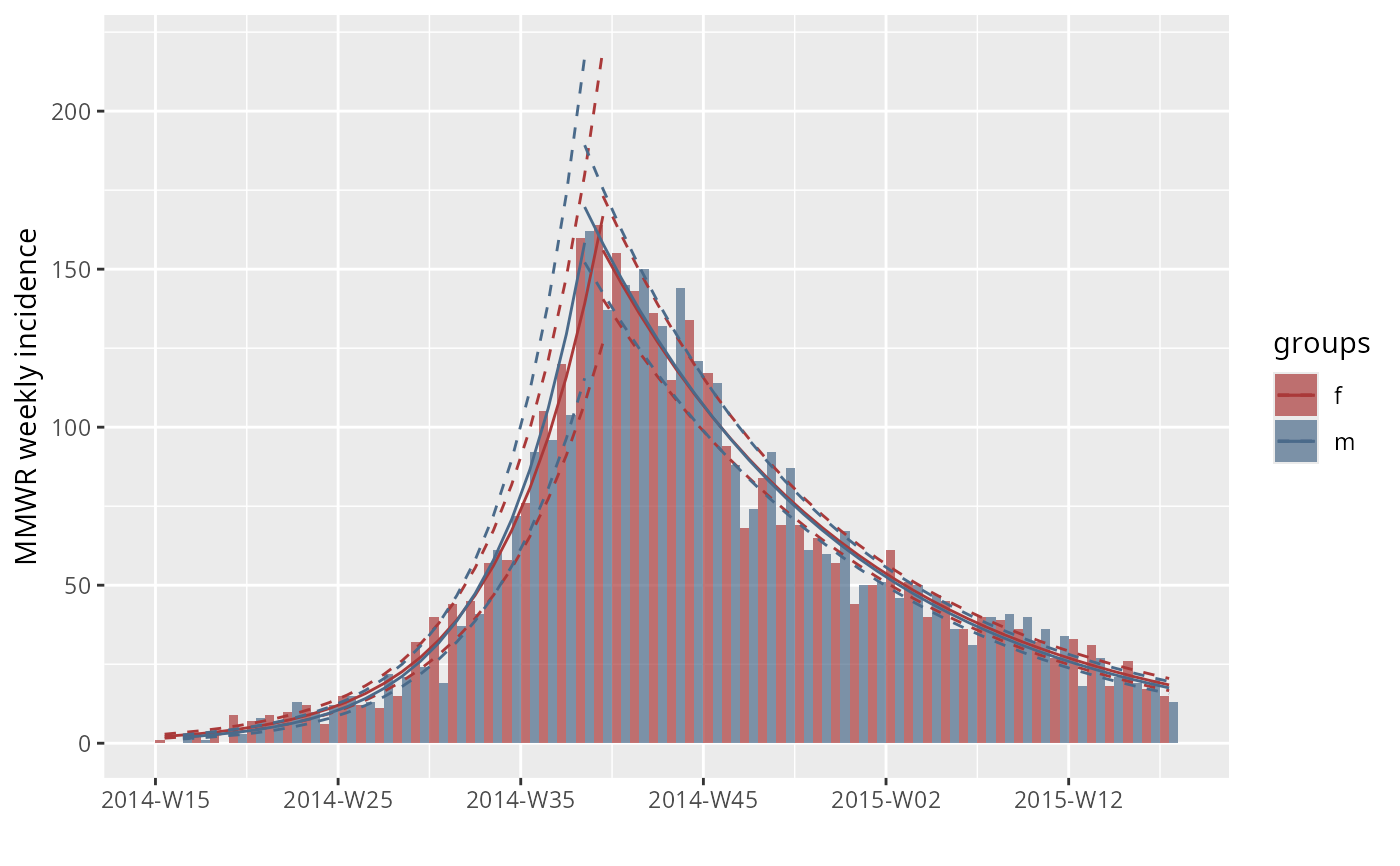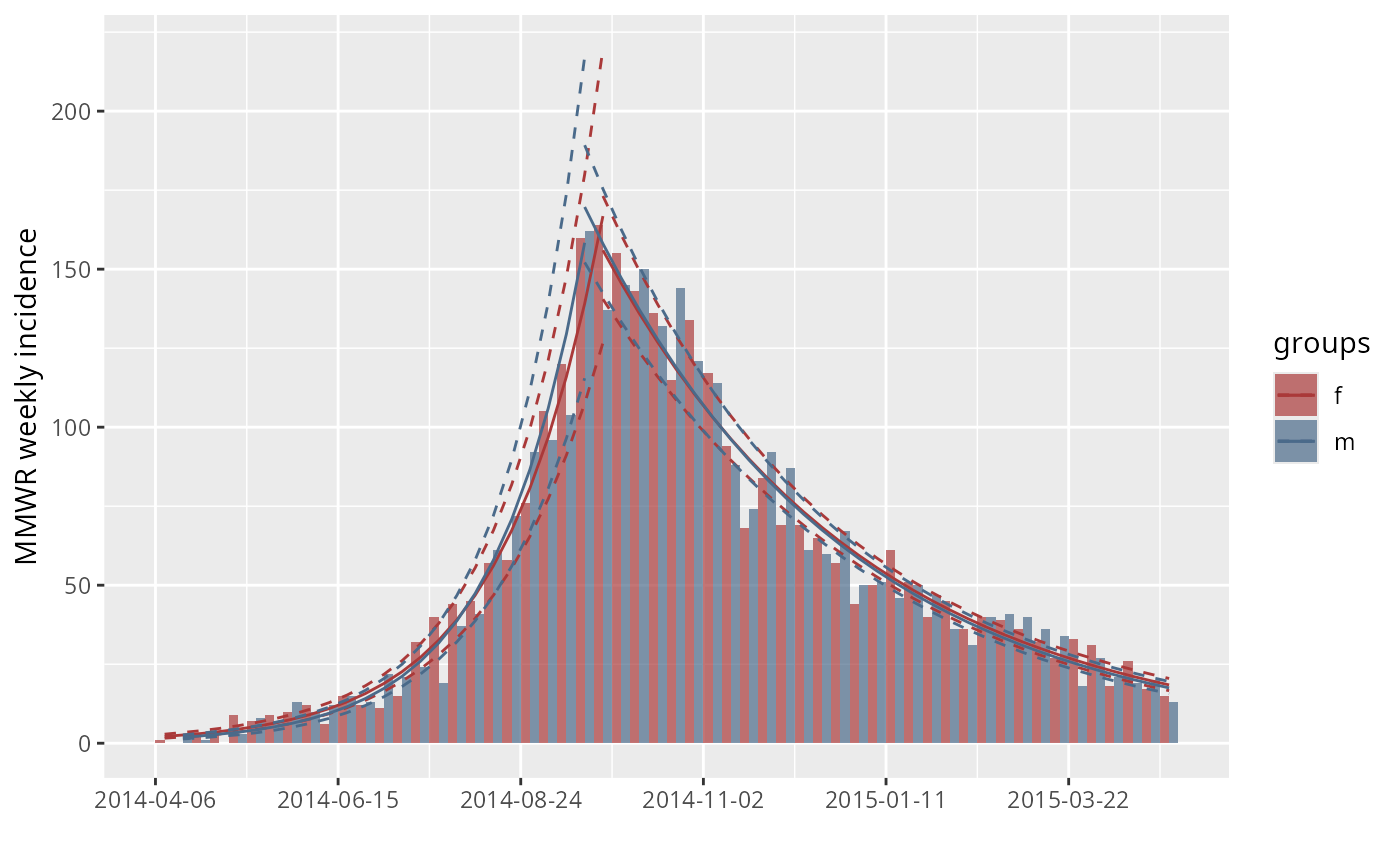This function is used to visualise the output of the incidence() function using the package ggplot2. #'

# S3 method for incidence
plot(
x,
...,
fit = NULL,
stack = is.null(fit),
color = "black",
border = NA,
col_pal = incidence_pal1,
alpha = 0.7,
xlab = "",
ylab = NULL,
labels_week = !is.null(x$weeks), labels_iso = !is.null(x$isoweeks),
show_cases = FALSE,
n_breaks = 6
)

# S3 method for incidence_fit
plot(x, ...)

# S3 method for incidence_fit_list
plot(x, ...)

scale_x_incidence(x, n_breaks = 6, labels_week = TRUE, ...)

make_breaks(x, n_breaks = 6L, labels_week = TRUE)

x An incidence object, generated by the function incidence(). arguments passed to ggplot2::scale_x_date(), ggplot2::scale_x_datetime(), or ggplot2::scale_x_continuous(), depending on how the date element is stored in the incidence object. An 'incidence_fit' object as returned by fit(). A logical indicating if bars of multiple groups should be stacked, or displayed side-by-side. The color to be used for the filling of the bars; NA for invisible bars; defaults to "black". The color to be used for the borders of the bars; NA for invisible borders; defaults to NA. The color palette to be used for the groups; defaults to incidence_pal1. See incidence_pal1() for other palettes implemented in incidence. The alpha level for color transparency, with 1 being fully opaque and 0 fully transparent; defaults to 0.7. The label to be used for the x-axis; empty by default. The label to be used for the y-axis; by default, a label will be generated automatically according to the time interval used in incidence computation. a logical value indicating whether labels x axis tick marks are in week format YYYY-Www when plotting weekly incidence; defaults to TRUE. (deprecated) This has been superceded by labels_iso. Previously:a logical value indicating whether labels x axis tick marks are in ISO 8601 week format yyyy-Www when plotting ISO week-based weekly incidence; defaults to be TRUE. if TRUE (default: FALSE), then each observation will be colored by a border. The border defaults to a white border unless specified otherwise. This is normally used outbreaks with a small number of cases. Note: this can only be used if stack = TRUE the ideal number of breaks to be used for the x-axis labeling An existing incidence plot. ## Value • plot() a ggplot2::ggplot() object. • make_breaks() a two-element list. The "breaks" element will contain the evenly-spaced breaks as either dates or numbers and the "labels" element will contain either a vector of weeks OR a ggplot2::waiver() object. • scale_x_incidence() a ggplot2 "ScaleContinuous" object. ## Details • plot() will visualise an incidence object using ggplot2 • make_breaks() calculates breaks from an incidence object that always align with the bins and start on the first observed incidence. • scale_x_incidence() produces and appropriate ggplot2 scale based on an incidence object. ## See also The incidence() function to generate the 'incidence' objects. ## Examples  if(require(outbreaks) && require(ggplot2)) { withAutoprint({ onset <- outbreaks::ebola_simlinelist$date_of_onset ## daily incidence inc <- incidence(onset) inc plot(inc) ## weekly incidence inc.week <- incidence(onset, interval = 7) inc.week plot(inc.week) # default to label x axis tick marks with isoweeks plot(inc.week, labels_week = FALSE) # label x axis tick marks with dates plot(inc.week, border = "white") # with visible border ## use group information sex <- outbreaks::ebola_sim$linelist$gender inc.week.gender <- incidence(onset, interval = "1 epiweek", groups = sex) plot(inc.week.gender) plot(inc.week.gender, labels_week = FALSE) ## show individual cases at the beginning of the epidemic inc.week.8 <- subset(inc.week.gender, to = "2014-06-01") p <- plot(inc.week.8, show_cases = TRUE, border = "black") p ## update the range of the scale lim <- c(min(get_dates(inc.week.8)) - 7*5, aweek::week2date("2014-W50", "Sunday")) lim p + scale_x_incidence(inc.week.gender, limits = lim) ## customize plot with ggplot2 plot(inc.week.8, show_cases = TRUE, border = "black") + theme_classic(base_size = 16) + theme(axis.text.x = element_text(angle = 90, hjust = 1, vjust = 0.5)) ## adding fit fit <- fit_optim_split(inc.week.gender)$fit
plot(inc.week.gender, fit = fit)
plot(inc.week.gender, fit = fit, labels_week = FALSE)

})}#> > onset <- outbreaks::ebola_sim$linelist$date_of_onset
#> > inc <- incidence(onset)
#> > inc
#> <incidence object>
#> [5888 cases from days 2014-04-07 to 2015-04-30]
#>
#> $counts: matrix with 389 rows and 1 columns #>$n: 5888 cases in total
#> $dates: 389 dates marking the left-side of bins #>$interval: 1 day
#> $timespan: 389 days #>$cumulative: FALSE
#>
#> > plot(inc)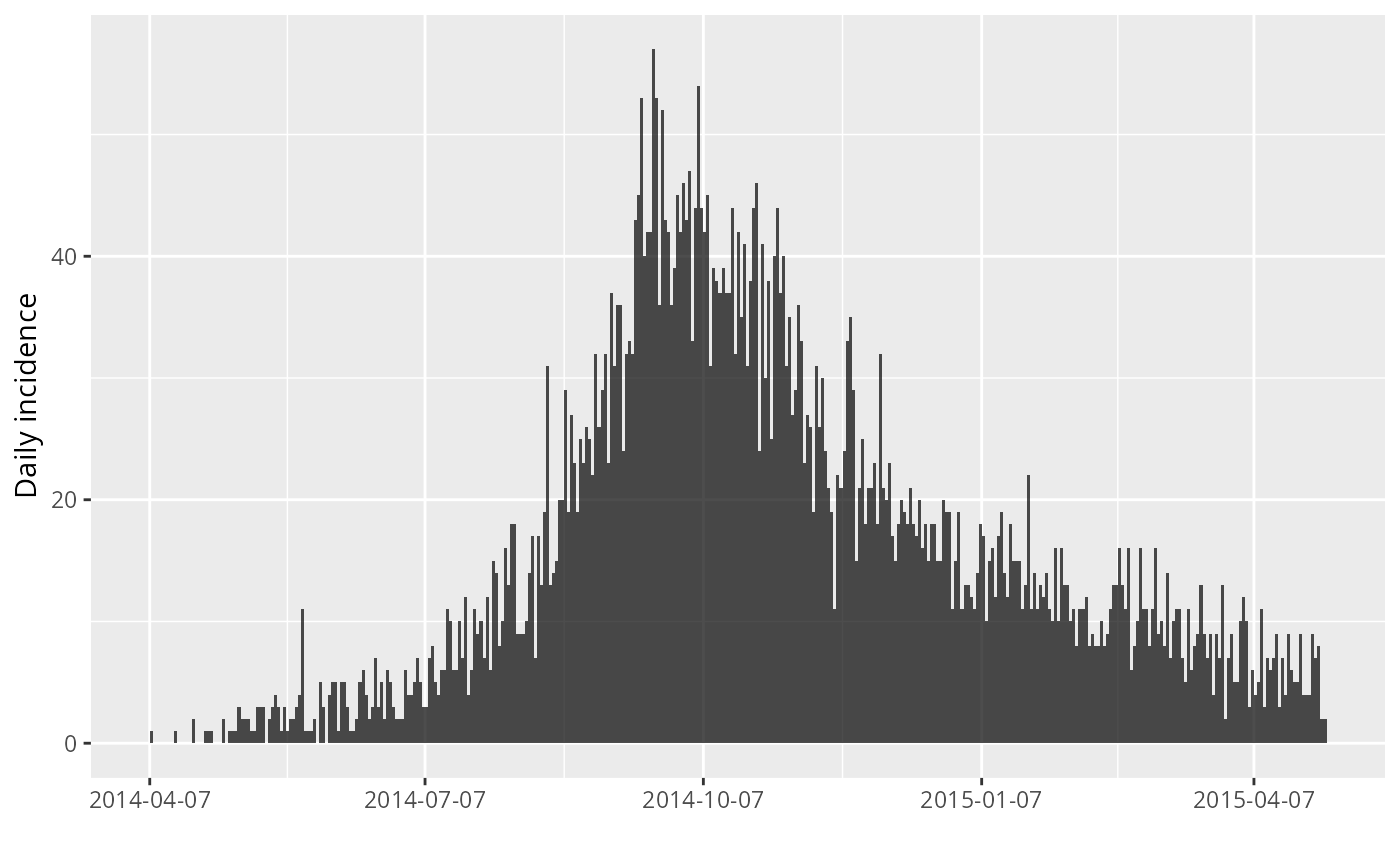#> > inc.week <- incidence(onset, interval = 7)
#> > inc.week
#> <incidence object>
#> [5888 cases from days 2014-04-07 to 2015-04-27]
#> [5888 cases from ISO weeks 2014-W15 to 2015-W18]
#>
#> $counts: matrix with 56 rows and 1 columns #>$n: 5888 cases in total
#> $dates: 56 dates marking the left-side of bins #>$interval: 7 days
#> $timespan: 386 days #>$cumulative: FALSE
#>
#> > plot(inc.week)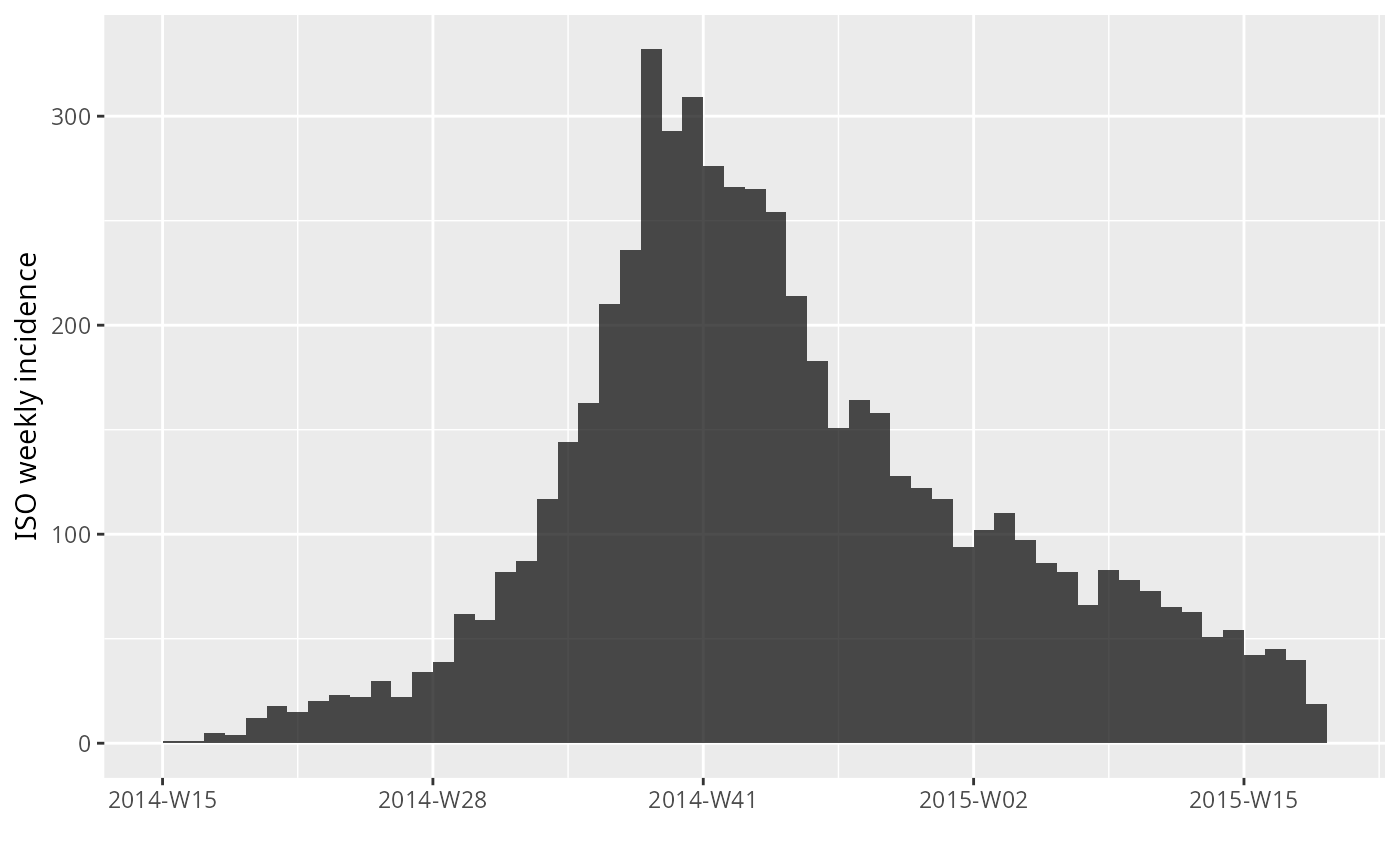#> > plot(inc.week, labels_week = FALSE)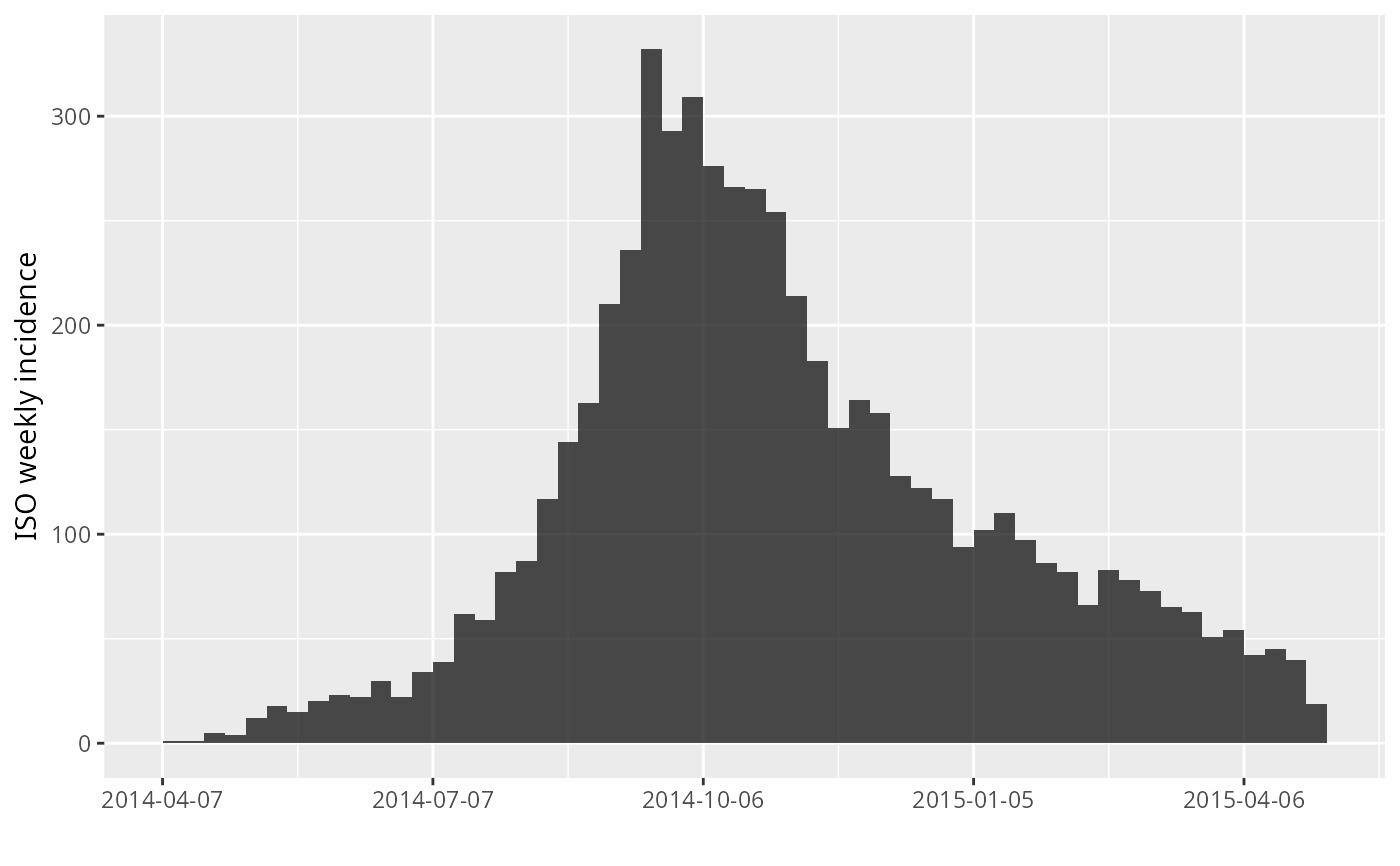#> > plot(inc.week, border = "white")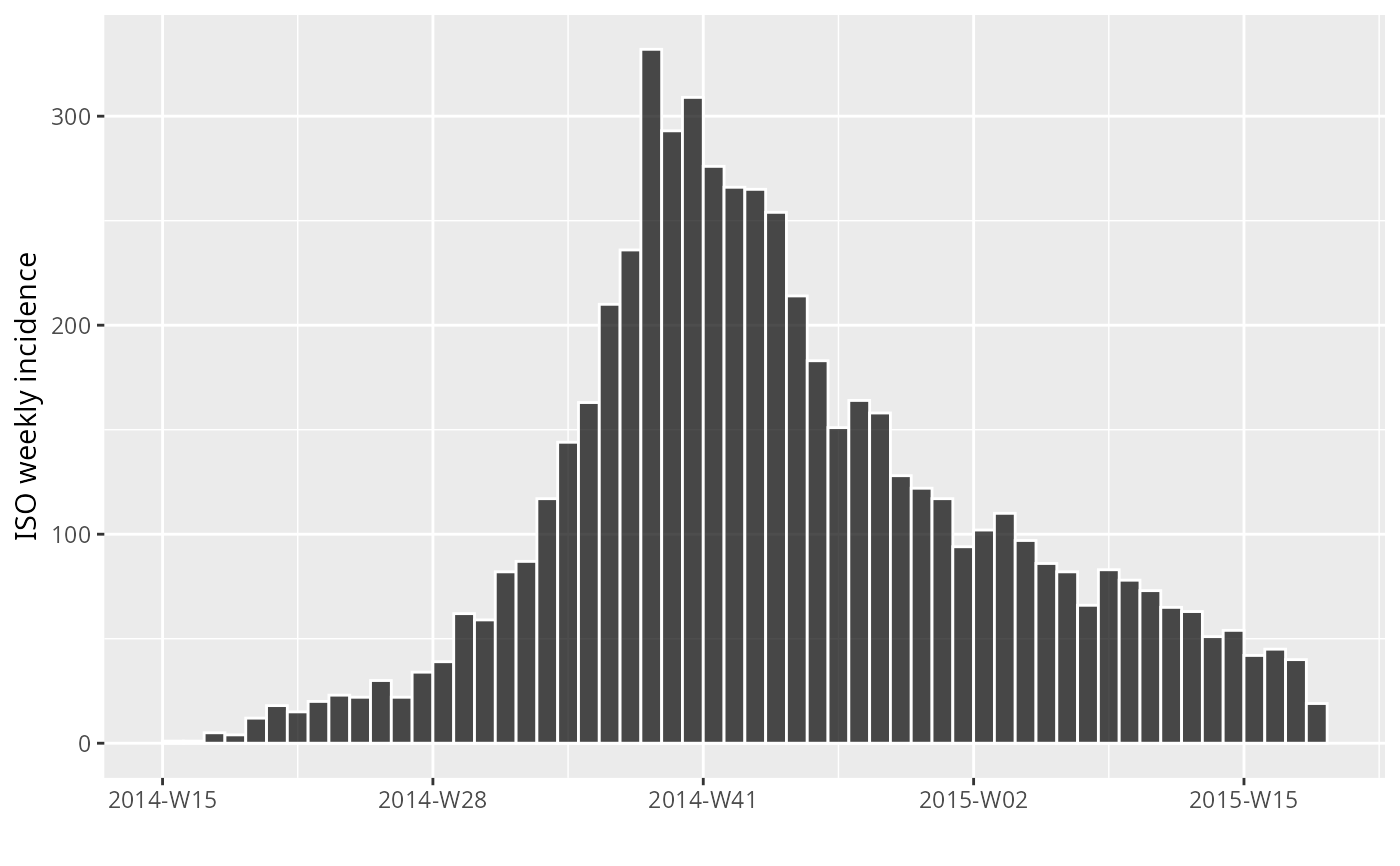#> > sex <- outbreaks::ebola_sim$linelist$gender
#> > inc.week.gender <- incidence(onset, interval = "1 epiweek", groups = sex)
#> > plot(inc.week.gender)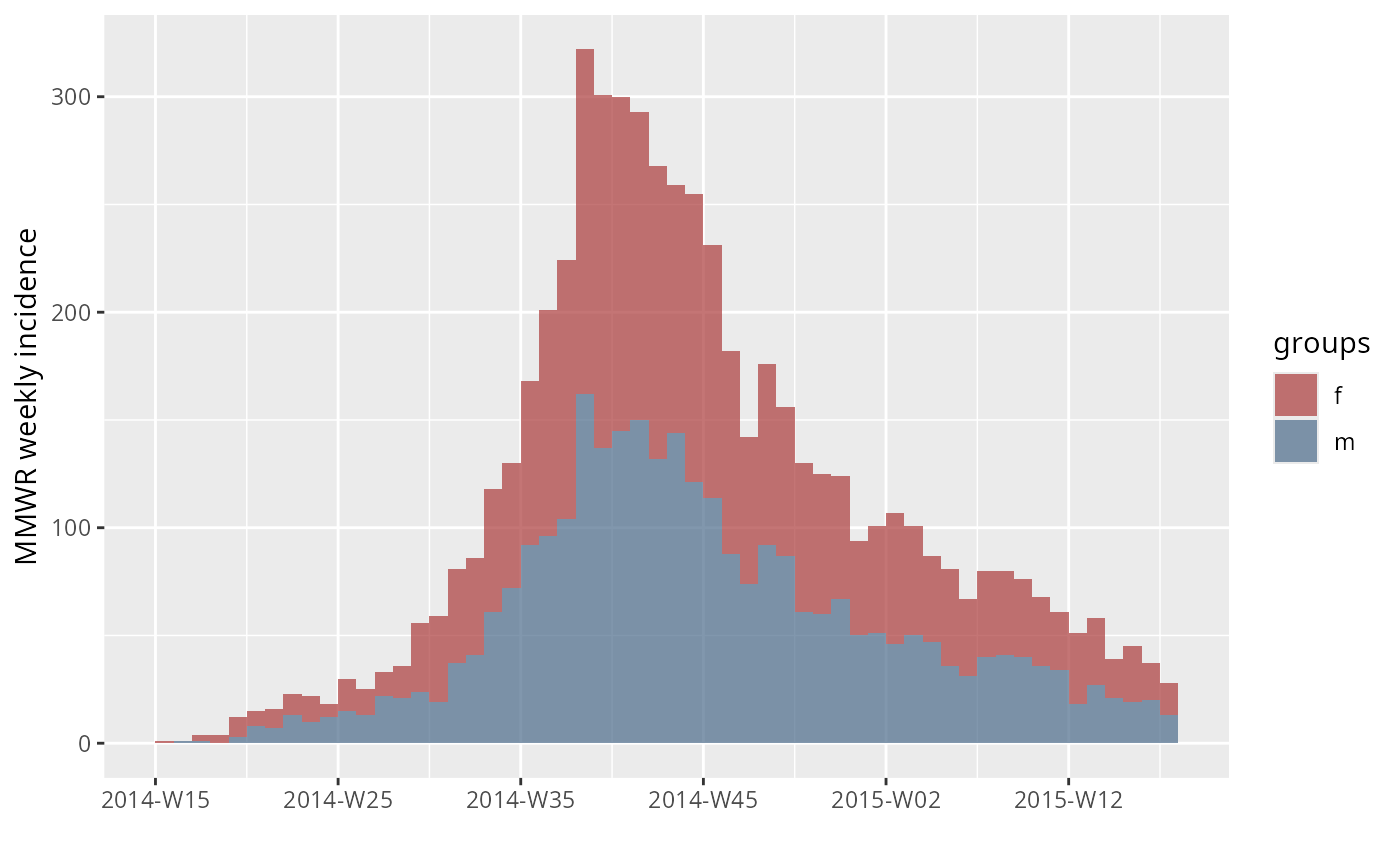#> > plot(inc.week.gender, labels_week = FALSE)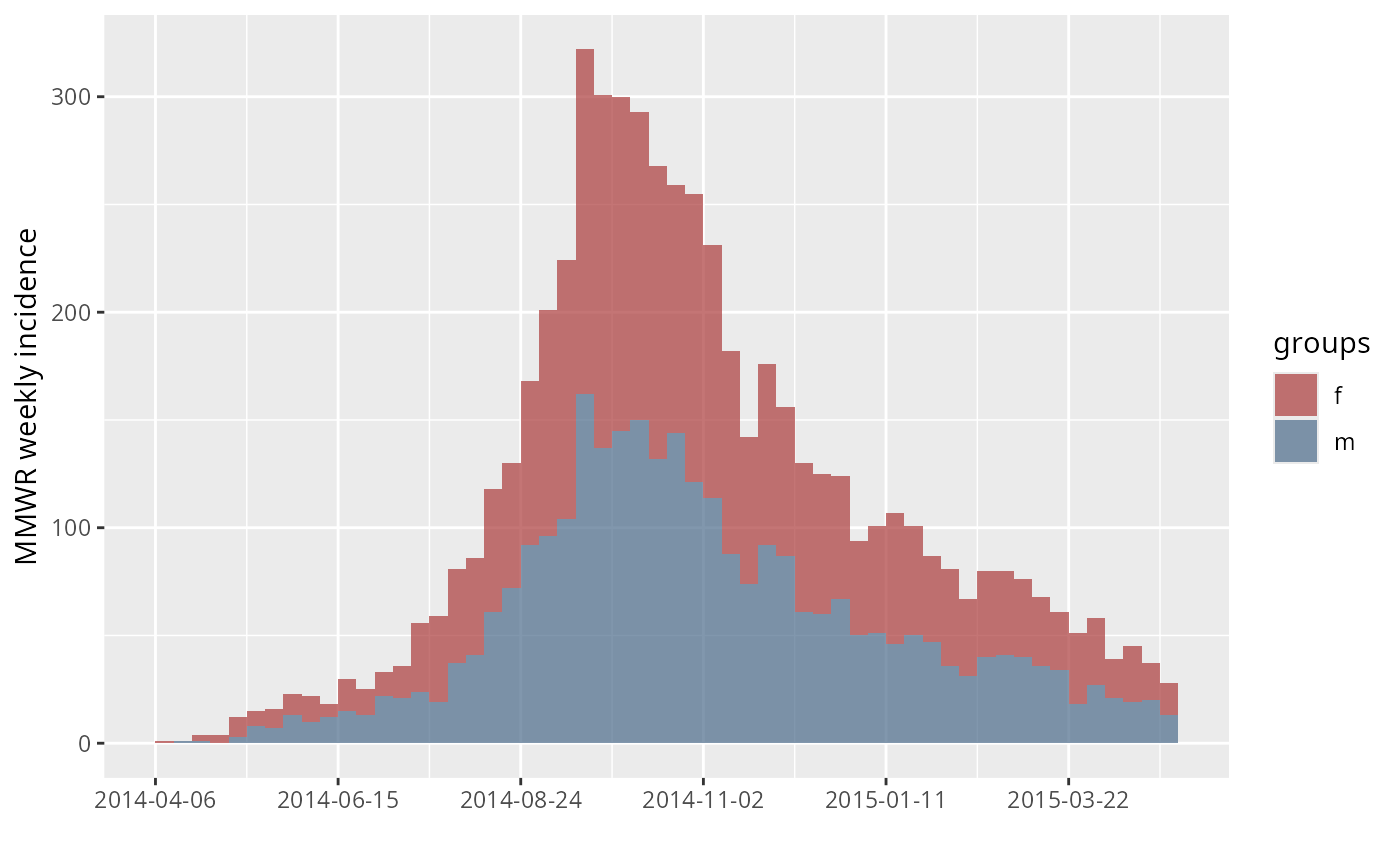#> > inc.week.8 <- subset(inc.week.gender, to = "2014-06-01")
#> > p <- plot(inc.week.8, show_cases = TRUE, border = "black")
#> > p#> > lim <- c(min(get_dates(inc.week.8)) - 7 * 5, aweek::week2date("2014-W50",
#> +     "Sunday"))
#> > lim
#>  "2014-03-02" "2014-12-07"
#> > p + scale_x_incidence(inc.week.gender, limits = lim)#> Scale for 'x' is already present. Adding another scale for 'x', which will
#> replace the existing scale.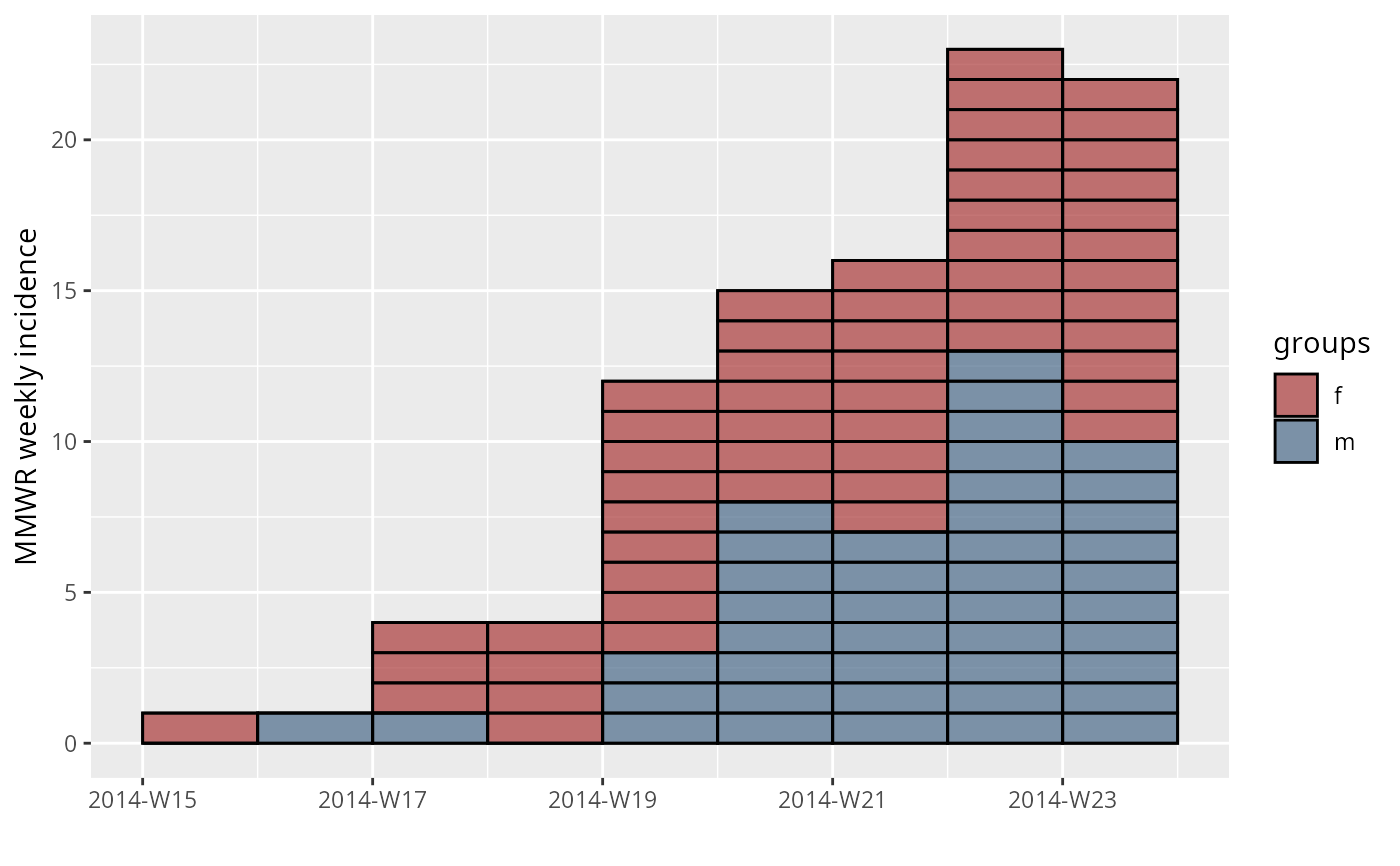#> > plot(inc.week.8, show_cases = TRUE, border = "black") + theme_classic(base_size = 16) +
#> +     theme(axis.text.x = element_text(angle = 90, hjust = 1, vjust = 0.5))#> > fit <- fit_optim_split(inc.week.gender)\$fit
#> > plot(inc.week.gender, fit = fit)#> Scale for 'colour' is already present. Adding another scale for 'colour',
#> which will replace the existing scale.#> Scale for 'colour' is already present. Adding another scale for 'colour',
#> which will replace the existing scale.#> Scale for 'colour' is already present. Adding another scale for 'colour',
#> which will replace the existing scale.#> Scale for 'colour' is already present. Adding another scale for 'colour',
#> which will replace the existing scale.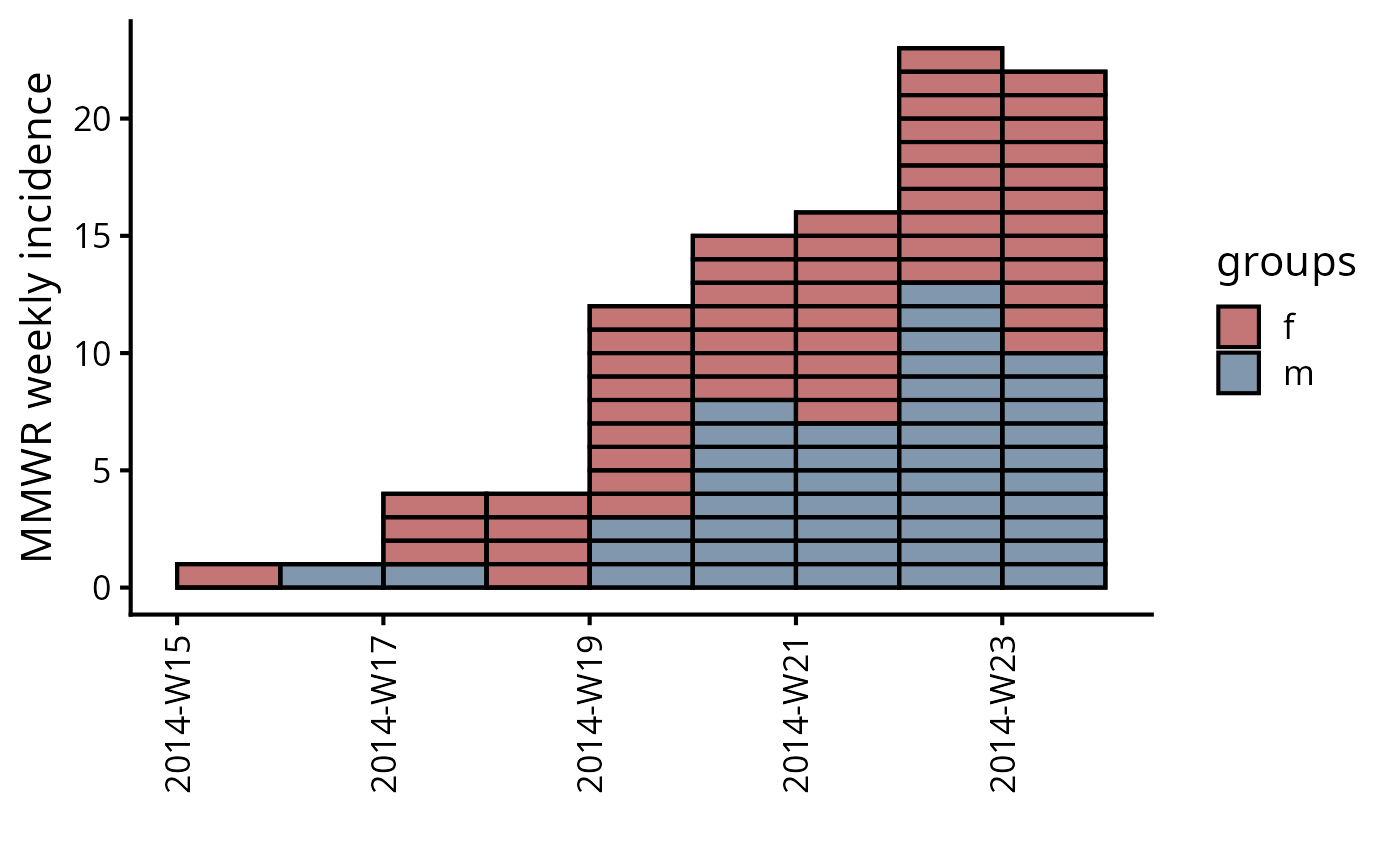#> > plot(inc.week.gender, fit = fit, labels_week = FALSE)#> Scale for 'colour' is already present. Adding another scale for 'colour',
#> which will replace the existing scale.#> Scale for 'colour' is already present. Adding another scale for 'colour',
#> which will replace the existing scale.#> Scale for 'colour' is already present. Adding another scale for 'colour',
#> which will replace the existing scale.#> Scale for 'colour' is already present. Adding another scale for 'colour',
#> which will replace the existing scale.